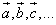In the plane euclidean geometry,

• given two distinct points A and B,
• given the straight line (which is unique) through A and B,
• given the direction from A to B so that we can say that A precedes B,

the segment AB is the set of the points P such that A is equal to or precedes P and P is equal to or precedes B: A≤P≤B.

Usually in the plane euclidean geometry the direction of a segment is not considered: the only relevant property of a segment is its length, that is its magnitude, which doesn't depend upon its orientation.

But many properties of the natural phenomena are characterized not only by their magnitude, but also by their line of action and by the direction on this line.

For example, to describe the displacement of an airplane on a geographical chart, it isn't sufficient to say that the plane has covered 1000 miles: we need to specificate the line of the fly, for instance W-E, and the direction of the fly, for instance from E to W.

Displacements and other properties with the same attributes are said vectorial quantities. To efficiently represent such quantities we can use oriented segments drawn as arrows, in which, given a suitable scale, the length is proportional to the magnitude, the line is the line of action and the head gives the direction. These arrows are said geometric vectors. In this note, vector means always geometric vector.

Usually vectors are denoted by boldface latin letters or by latin letters with an arrow written on top, so the following notations are equivalent

a, b, c,...For example, to represent on a geographical map, such that 1cm corresponds to 1km, the trip of a car which has moved 4km toward W, we'll draw a 4cm long horizontal arrow with the head on the leftmost end. The other end is the 'tail' of the vector.

It is important to emphasize that the displacement doesn't depend from the starting or ending point. If a car starts from Paris and another car starts from London and both move toward W covering 100km, at the end of the trip they we'll be in different places, but they we'll have the same displacement.

In general, we can say that parallel, equally oriented and equally long vectors are equivalent. Therefore a vector can be freely translated in the space.